# 14.3 Discussion and Exercises

The external memory model of computation was introduced by Aggarwal and Vitter . It is sometimes also called the I/O model or the disk access model.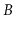-Trees are to external memory searching what binary search trees are to internal memory searching.-trees were introduced by Bayer and McCreight  in 1970 and, less than ten years later, the title of Comer's ACM Computing Surveys article referred to them as ubiquitous .

Like binary search trees, there are many variants of-Trees, including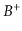-trees,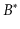-trees, and counted-trees.-trees are indeed ubiquitous and are the primary data structure in many file systems, including Apple's HFS+, Microsoft's NTFS, and Linux's Ext4; every major database system; and key-value stores used in cloud computing. Graefe's recent survey  provides a 200+ page overview of the many modern applications, variants, and optimizations of-trees.-trees implement the SSet interface. If only the USet interface is needed, then external memory hashing could be used as an alternative to-trees. External memory hashing schemes do exist; see, for example, Jensen and Pagh . These schemes implement the USet operations in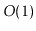expected time in the external memory model. However, for a variety of reasons, many applications still use-trees even though they only require USet operations.

One reason-trees are such a popular choice is that they often perform better than their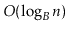running time bounds suggest. The reason for this is that, in external memory settings, the value ofis typically quite large--in the hundreds or even thousands. This means that 99% or even 99.9% of the data in a-tree is stored in the leaves. In a database system with a large memory, it may be possible to cache all the internal nodes of a-tree in RAM, since they only represent 1% or 0.1% of the total data set. When this happens, this means that a search in a-tree involves a very fast search in RAM, through the internal nodes, followed by a single external memory access to retrieve a leaf.

Exercise 14..1   Show what happens when the keys 1.5 and then 7.5 are added to the-tree in Figure 14.2.

Exercise 14..2   Show what happens when the keys 3 and then 4 are removed from the-tree in Figure 14.2.

Exercise 14..3   What is the maximum number of internal nodes in a-tree that stores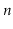keys (as a function ofand)?

Exercise 14..4   The introduction to this chapter claims that-trees only need an internal memory of size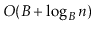. However, the implementation given here actually requires more memory.
1. Show that the implementation of theand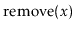methods given in this chapter use an internal memory proportional to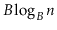.
2. Describe how these methods could be modified in order to reduce their memory consumption to.

Exercise 14..5   Draw the credits used in the proof of Lemma 14.1 on the trees in Figures 14.6 and 14.7. Verify that (with three additional credits) it is possible to pay for the splits, merges, and borrows and maintain the credit invariant.

Exercise 14..6   Design a modified version of a-tree in which nodes can have anywhere fromup to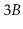children (and hence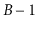up to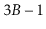keys). Show that this new version of-trees performs only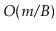splits, merges, and borrows during a sequence of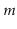operations. (Hint: For this to work, you will have to be more agressive with merging, sometimes merging two nodes before it is strictly necessary.)

Exercise 14..7   In this exercise, you will design a modified method of splitting and merging in-trees that asymptotically reduces the number of splits, borrows and merges by considering up to three nodes at a time.
1. Letbe an overfull node and let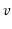be a sibling immediately to the right of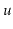. There are two ways to fix the overflow at:
1.can give some of its keys to; or
2.can be split and the keys ofandcan be evenly distributed among,, and the newly created node,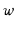.
Show that this can always be done in such a way that, after the operation, each of the (at most 3) affected nodes has at least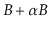keys and at most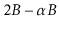keys, for some constant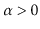.
2. Letbe an underfull node and letandbe siblings ofThere are two ways to fix the underflow at:
1. keys can be redistributed among,, and; or
2.,, andcan be merged into two nodes and the keys of,, andcan be redistributed amongst these nodes.
Show that this can always be done in such a way that, after the operation, each of the (at most 3) affected nodes has at leastkeys and at mostkeys, for some constant.
3. Show that, with these modifications, the number of merges, borrows, and splits that occur duringoperations is.

Exercise 14..8   A-tree, illustrated in Figure 14.11 stores every key in a leaf and keeps its leaves stored as a doubly-linked list. As usual, each leaf stores betweenand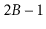keys. Above this list is a standard-tree that stores the largest value from each leaf but the last.
1. Describe fast implementations of,, andin a-tree.
2. Explain how to efficiently implement themethod, that reports all values greater than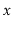and less than or equal to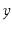, in a-tree.
3. Implement a class, BPlusTree, that implements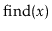,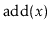,, and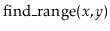.
4.-trees duplicate some of the keys because they are stored both in the-tree and in the list. Explain why this duplication does not add up to much for large values of.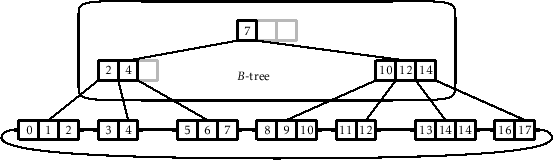opendatastructures.org